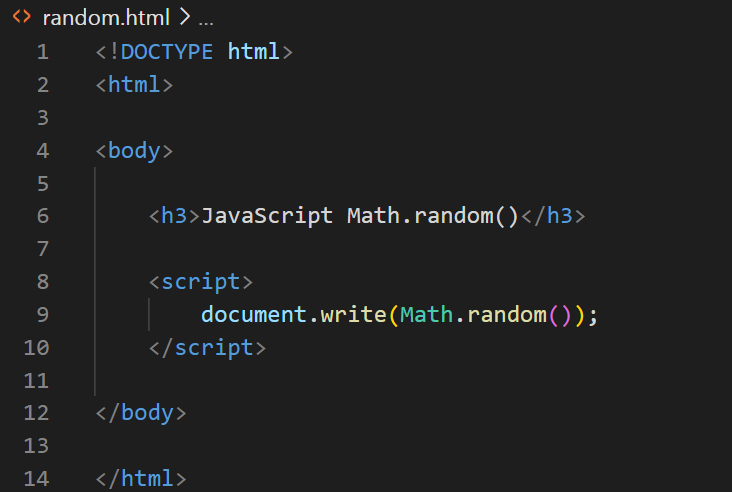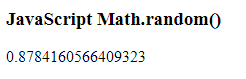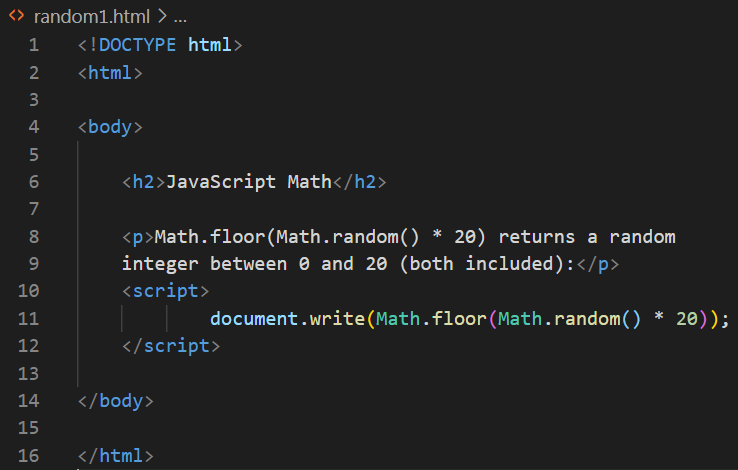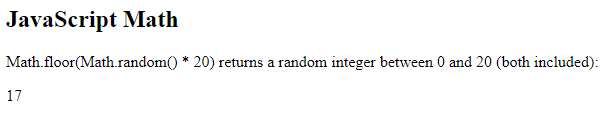JavaScript Random | JS random | Random Number Generator Javascript

# JavaScript Random

JavaScript Random
In this tutorial, you will learn about random() is as follows:
• The Random Method returns number between 0 (inclusive) and 1 (exclusive)
• Math.random()
• There are no parameters for  method random().
ExampleOutputShifting and Scaling
The range of the values produced directly by a random variable is often different from what is needed in a specific application.
e.g.,
• Dice
• Coin Tossing
Math.floor(1+ Math.random()0*6)
Here, Math.random is multiplied with 6, so that we get a range from 0.0 6.0. This is called scaling.
Adding 1(here) to the obtained value is called shifting.
Math.floor() is used to determine the doset integer not greater than the argument value
• E.g., Math.floor(6.75) is 6 and Math.floor(1.89) is 1
Example1- ProgramDisplaying Random Images
Web content that varies randomly can add dynamic, interesting effects to a page.